Rolling N-gon Pencil

Umut Eser October 20, 2008

(Submitted as coursework for Physics 210, Stanford University, Autumn 2007)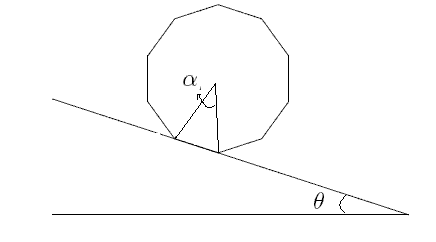Fig. 1: N-gon prism on an inclined plane.

When I was in high school, I was preparing to the 33rd International Physics Olympiad (IPhO). At that time (and still indeed) the most enjoyable problems to solve are those which are not obvious to conceive but can be demonstrated easily and have simple solutions. The rolling pencil problem is one of them. The 1st problem of the IPhO 29th was asking to calculate the terminal velocity of a hexagonal prism rolling on an inclined plane. Being a high school student, I thought it was a good didactic question to fight with. An instinct was forcing me to deal with general case (i.e. N-gon prisms) instead of just hexagonal pencils so that I could check what happens if the pencil were cylinder.

To deal with the problem, first, we should assume that there is no sliding, therefore no kinetic friction. Besides, the prism does not loose the contact. Every time one edge of the n-gon pencil strikes to the plane, it loses some energy because of the inelasticity of the pencil.

In order to understand the physics and find the limit velocity, lets consider the angle of inclination to be θ (cf. Fig. 1). The condition limiting the velocity is that the kinetic energy gain during one step of rotation dissipates via the collision of the edge with the surface proportional to the energy before collision.

The pencil, which is initially at rest, starts rolling with the following equation of motion:This is called Mathieu Equation and does not have an analytic solution. It is therefore useful to consider the energy equations to find the limit velocity. The energy gain during one step of rotation (i.e. 1/N of full revolution) is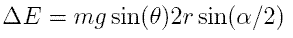Lets say the elasticity coefficient is ε. Then the energy right after the collision can be written as: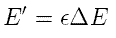Right after the second collision, the energy becomes: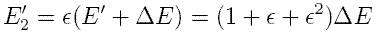If we continue this iteration, we'll find that the nth step energy of the pencil is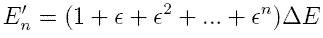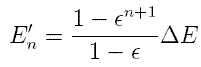The asymptotic value of this function goes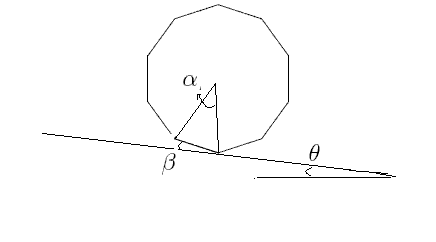Fig. 2: The N-gon prism rotated by β.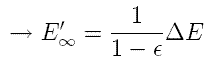During one step of rotation, the kinetic enrgy changes. The vertical position of the center of mass before rotating is z0 = rcos(θ - α/2). At an angle of rotation β , the position becomes z = r cos(θ - α/2 + β). Therefore, the average value of the energy can be found by taking the average integral over the rotation angle α.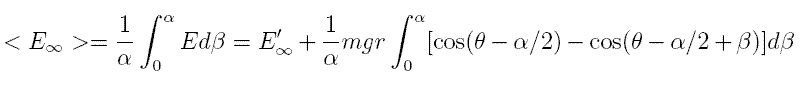After evaluating the integral, the asymptotic energy can be found as: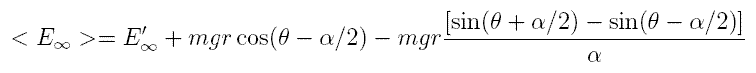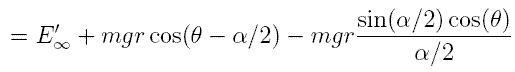If ω is the average angular velocity, then the energy can be written as: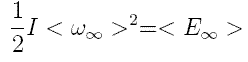where I is the moment of inertia around the contact point. Using these equations, the average limit angular velocity and the average limit velocity can be expressed as follows: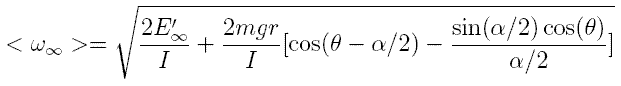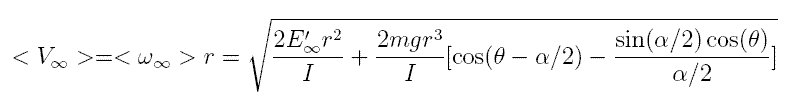Recall that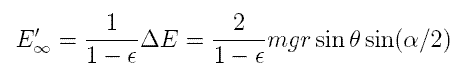For high values of the side number (that means small values of α) the moment of inertia aproaches to 3/2 mr2. If we plug the moment of inertia and the energy equation into the velocity expression, we find: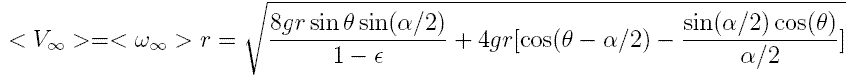The interesting part of this result is, if α goes zero, the limit velocity also goes zero regardless of θ which is nonsense. Therefore, the assumptions made at the begining are no longer valid. If the pencil reaches a certain velocity, it will start sliding and the rolling friction applies. For an N-gon prism, the sliding would appear only a small part of stepwise rotation of which duration depends on the velocity. Therefore, the kinetic friction of the cylindirical pencil cannot have just constant value. Instead it may polynomially dependent on velocity.

Another suspicious assumption is to consider the ε being independent of the side number. That is to say, if N goes infinity and the prism approaches to cylinder, the elasticity coefficient should approach to one since the impulsive force is no longer much more greater than the continuous ones.

© 2007 U. Eser. The author grants permission to copy, distribute and display this work in unaltered form, with attribution to the author, for noncommercial purposes only. All other rights, including commercial rights, are reserved to the author.

References

1st Problem of the 29th International Physics Olympiad (July 4, 1998),